# QSO points

First phase is computing points for every QSO. Tucnak uses scoring methods from Taclog but some are unimplemented or can be buggy. Here are described only tested ones.

## 1 Standard IARU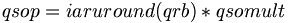$qsop = iaruround(qrb) * qsomult$

The iaruround function computes points from QRB (distance between stations).

## 2 QSO multipliers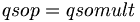$qsop = qsomult$

## 4 WWL ring

Points are computed by big WWL (like JN69). Own locator (JN69) brings 2 points, neighbors (JO50, JO60, JO70, JN59, JN79, JN58, JN68 JN78) are 3 point etc.

JO50 JO60 JO70
JN59 JN69 JN79
JN58 JN68 JN78


## 12 RDGB DC

Same like 1 but EXC multipliers can be counted:

• 6 times for BT
• 3 times for AB, DD, DG, EH, FK, GS, HS, IV, KA, KY, PA, PH, TD, ZE
• 1 times for all others

## 13 Continent

For CQ WW DX Contest. QSO points are:

• 0 for same DXCC
• 1 for same continent
• 2 for same continent within north America
• 3 for other continent

## 14 Continent + Band

For CQ WW WPX Contest. QSO points are:

• 1 for same DXCC
• 1 for same continent
• 2 for same continent within north America
• 3 for other continent

Points for lower bands (160, 80 and 40m) are multiplied by 2 (2, 2, 4, 6).

## 15 ARRL VHF Contest

• 1 for 144 MHz and bellow
• 2 for 222 MHz and 432 MHz
• 3 for 902 MHz and 1,3 GHz
• 4 for 2,3 GHz and above

## 16 Championat de France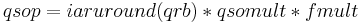$qsop = iaruround(qrb) * qsomult * fmult$

Where qsomult is:

• 1 for 144 MHz
• 3 for 432 MHz
• 5 for 1,3 GHz
• 10 for 2,3 GHz and above

Fmult is 4 for stations from F, TK, TM and 1 for others.

# Total points

## 1 Multiplicative

For most contests like standard IARU.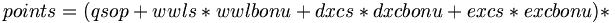$points = ( qsop + wwls * wwlbonu + dxcs * dxcbonu + excs * excbonu ) *$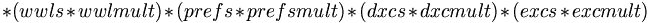$* ( wwls * wwlmult ) * ( prefs * prefsmult ) * ( dxcs * dxcmult ) * ( excs * excmult )$$points = ( qsop + wwls * wwlbonu + dxcs * dxcbonu + excs * excbonu ) *$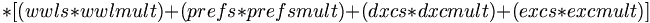$* [ ( wwls * wwlmult ) + ( prefs * prefsmult ) + ( dxcs * dxcmult ) + ( excs * excmult )]$

For CW WW DX Contest, multipliers are computed for all bands.$points = ( qsop + wwls * wwlbonu + dxcs * dxcbonu + excs * excbonu ) *$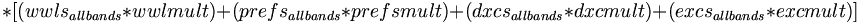$* [ ( wwls_{allbands} * wwlmult ) + ( prefs_{allbands} * prefsmult ) + ( dxcs_{allbands} * dxcmult ) + ( excs_{allbands} * excmult )]$

## 4 All bands together

For ARRL VHF Contest. Multipliers for all bands multipled by qso points.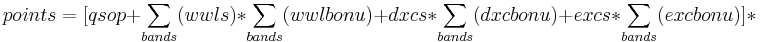$points = [ qsop + \sum_{bands}(wwls) * \sum_{bands}(wwlbonu) + dxcs * \sum_{bands}(dxcbonu) + excs * \sum_{bands}(excbonu) ] *$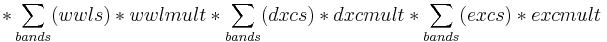$* \sum_{bands}( wwls ) * wwlmult * \sum_{bands}(dxcs) * dxcmult * \sum_{bands}(excs) * excmult$